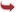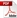International Journal of Scientific and Research Publications

#### IJSRP, Volume 3, Issue 8, August 2013 Edition [ISSN 2250-3153]Greedy Algorithm
Annu Malik, Anju Sharma, Mr. Vinod Saroha (Guide)
Abstract: This paper presents a survey on Greedy Algorithm. This discussion is centered on overview of Activity Selection Problem and Task Scheduling Problem . A greedy algorithm is an algorithm that follows the problem solving heuristic of making the locally optimal choice at each stage with the hope of finding a global optimum. In many problems, a greedy strategy does not in general produce an optimal solution, but nonetheless a greedy heuristic may yield locally optimal solutions that approximate a global optimal solution in a reasonable time.Greedy algorithms determine the minimum number of coins to give while making change. These are the steps a human would take to emulate a greedy algorithm to represent 36 cents using only coins with values {1, 5, 10, 20}. The coin of the highest value, less than the remaining change owed, is the local optimum. (Note that in general the change-making problem requires dynamic programming or integer programming to find an optimal solution; However, most currency systems, including the Euro and US Dollar, are special cases where the greedy strategy does find an optimum solution.)
[VIEW FULL PAPER][DOWNLOAD]

Reference this Research Paper (copy & paste below code):

Annu Malik, Anju Sharma, Mr. Vinod Saroha (Guide) (2018); Greedy Algorithm; Int J Sci Res Publ 3(8) (ISSN: 2250-3153). http://www.ijsrp.org/research-paper-0813.php?rp=P201564